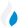•Trade Pi
•Trade
•Exchange
•Trade Pi
•Trade
•Exchange
\$1.08T
Total marketcap
\$79.8B
Total volume
40.91%
BTC dominance
•Trade Pi
•Trade
•Exchange

# cube to USD Exchange Rate - 1 Somnium Space CUBEs in USD

1.3719
• ETH 0.
Vol [24h]
\$75,429.67

## cube to USD converter

Exchange Pair Price 24h volume
Gemini CUBE/USD \$1.36 \$76.02K

## CUBE/USD Exchange Rate Overview

Name Ticker Price % 24h 24h high 24h low 24h volume
Somnium Space CUBEs cube \$1.35 -2.7006% \$1.44 \$1.3 \$145.55K

Selling 1 Somnium Space CUBEs cube you get 1.3719 USD.

Somnium Space CUBEs Nov 3, 2021 had the highest price, at that time trading at its all-time high of \$28.12.

454 days have passed since then, and now the price is 4.80% of the maximum.

Based on the table data, the CUBE vs USD exchange volume is \$75,429.67.

Using the calculator/converter on this page, you can make the necessary calculations with a pair of Somnium Space CUBEs to USD.

## Q&A

### What is the current CUBE to USD exchange rate?

Right now, the CUBE/USD exchange rate is 1.3719.

### What has been the Somnium Space CUBEs to USD trading volume in the last 24 hours?

Relying on the table data, the Somnium Space CUBEs to USD exchange volume is \$75,429.67.

### How can I calculate the amount of USD? / How do I convert my Somnium Space CUBEs to USD?

You can calculate/convert USD from CUBE to USD converter. Also, you can select other currencies from the drop-down list.

## CUBE to USD Сonversion Table

CUBE USD
0.3 CUBE = 0.4 USD
0.7 CUBE = 0.9 USD
1 CUBE = 1.3 USD
2 CUBE = 2.7 USD
4 CUBE = 5.4 USD
7 CUBE = 9.6 USD
20 CUBE = 27.4 USD
40 CUBE = 54.8 USD
80 CUBE = 109.7 USD
800 CUBE = 1,097.5 USD
8000 CUBE = 10,975.2 USD
80000 CUBE = 109,752 USD
800000 CUBE = 1,097,520 USD